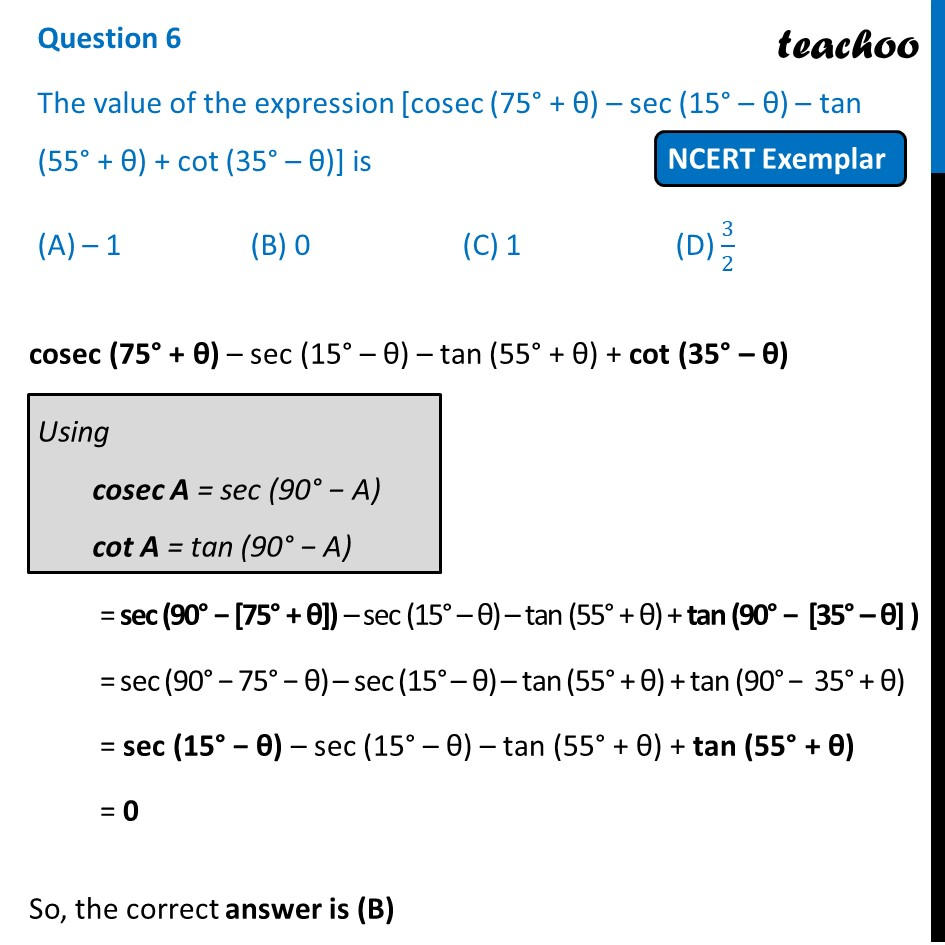## (A) – 1   (B) 0   (C) 1  (D) 3/21. Chapter 8 Class 10 Introduction to Trignometry (Term 1)
2. Serial order wise
3. NCERT Exemplar - MCQ

Transcript

Question 6 The value of the expression [cosec (75° + θ) – sec (15° – θ) – tan (55° + θ) + cot (35° – θ)] is (A) – 1 (B) 0 (C) 1 (D) 3/2 cosec (75° + θ) – sec (15° – θ) – tan (55° + θ) + cot (35° – θ) = sec (90° − [75° + θ]) – sec (15° – θ) – tan (55° + θ) + tan (90° − [35° – θ] ) = sec (90° − 75° − θ) – sec (15° – θ) – tan (55° + θ) + tan (90° − 35° + θ) = sec (15° − θ) – sec (15° – θ) – tan (55° + θ) + tan (55° + θ) = 0 So, the correct answer is (B)